### Flattening the impedance curve

A zobel network or an impedance equalization circuit is always a good idea when it comes to passive x-overs. Because impedance changes its value depending on frequency, it creates certain problems. Impedance will have a bump at resonance, and then it will slowly rise (as frequency increases), due to voice coil inductance.

Here is the thought process of why you should consider an impedance equalization circuit :

• The values of the components of the crossover network are dependent on the impedance of the speaker.
• If the impedance changes, so does the crossover frequency.
• In conclusion, if the impedance remains constant, the crossover frequency will keep its value, regardless of frequency.

#### Quick example :

To make a 1st order low pass filter for a mid-bass driver, all you need to do is to add an inductor in series with the speaker (more info here).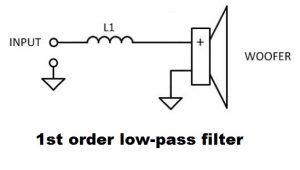The value of the inductor is calculated using this formula (for a Butterworth design):

L1 = Z / (2π * fC).

• L1 = inductance of the inductor (in Henries).
• Z = rated impedance of the speaker (in ohms).
• f= crossover frequency (in hertz).

Let’s say the speaker is 8 ohm and we want to cross it at 2000 Hz.

L1 = 8 / (2 * 3.1416 * 2000) = 0.63 mH.

So, if we add a 0.63 mH inductor in series with the speaker, we will attenuate all the upper frequencies by 6 dB / octave, starting with 2 kHz. This remains true, if the impedance value remains constant, which we know it doesn’t.

### Impedance vs frequency

Let’s use a real world speaker and make an impedance equalization circuit (also known as Zobel circuit / network) for it. The speaker I chose is SEAS Prestige CA 18 RNX (Amazon affiliate paid link). A 7″ mid-woofer, which will serve as an example on how to flatten the impedance curve.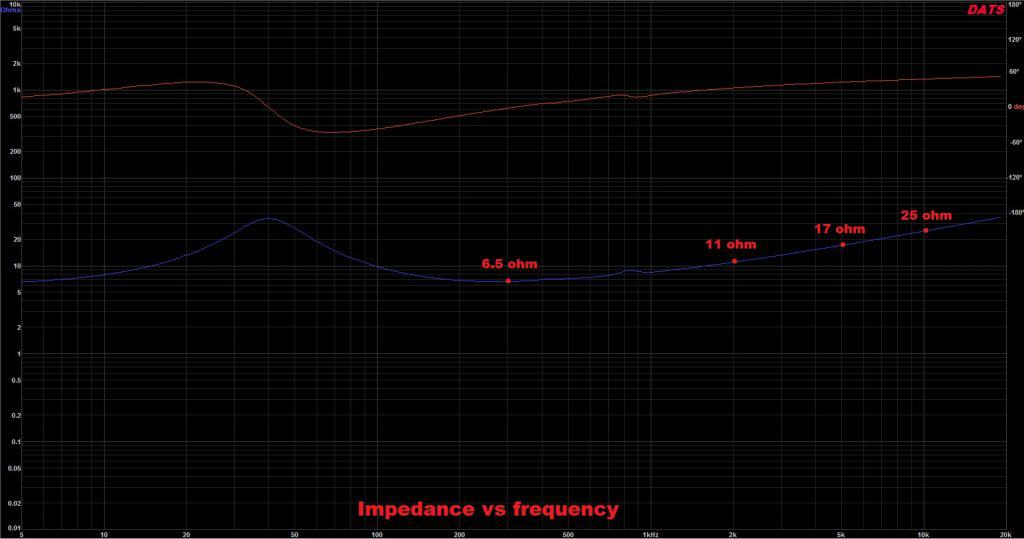The nominal impedance of this driver is 8 ohms. As you can see from the graph, the impedance rises steadily with frequency. As a result, this will interfere with the way the crossover network will work. To fix this, all we need to do is to check the zobel network calculator and implement the circuit.

### Impedance equalization circuit / Zobel network calculator

We all get intimidated by electrical circuits, don’t we? However, there is nothing to get concerned about, when it comes to a Zobel circuit. All you need is a resistor and a capacitor. That’s it!

Electrical diagram :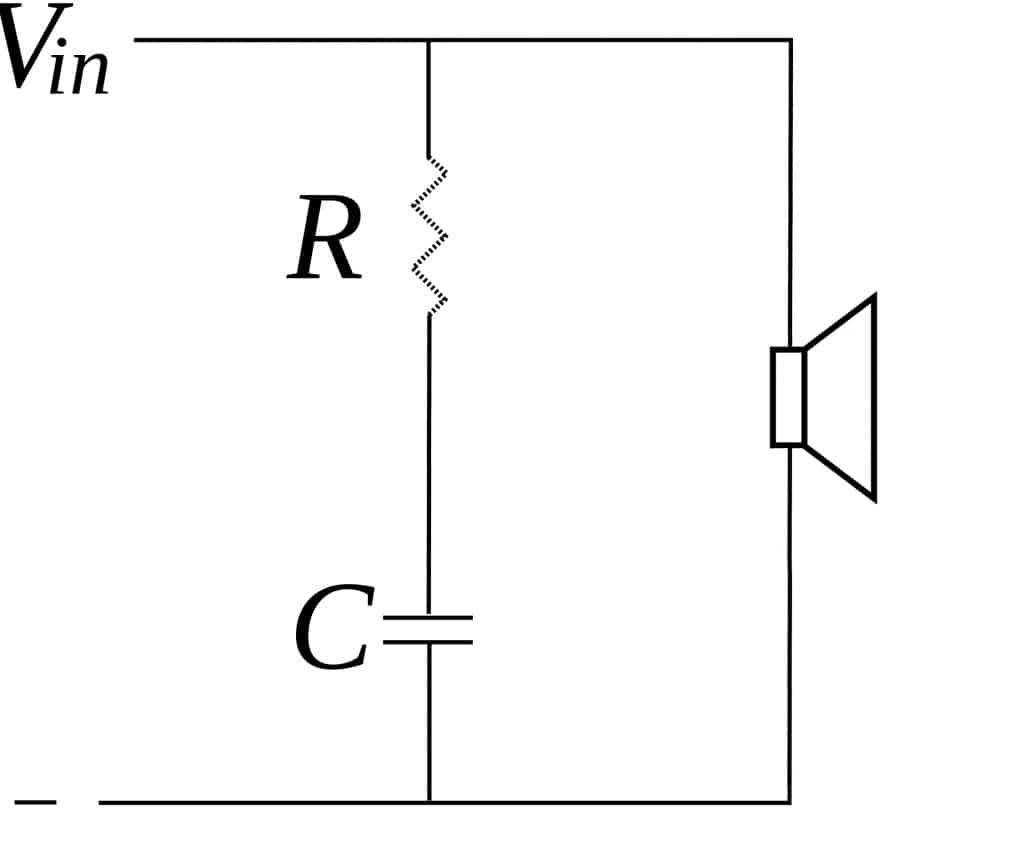To calculate the values of the electrical components, you just need some speaker parameters:

• R = 1.25 * Re
• C = Le / R2
• Re is the DC resistance of the speaker.
• Le is the inductance of the voice coil of the speaker.
• R and C are the values of the resistor and capacitor for the impedance equalization circuit.

### How to design loudspeakers - video courses

Let’s use these formulas for our SEAS CA 18 RNX speaker. You can find the spec sheet here. Anyway, we are extracting only the parameters we need : the DC resistance and the inductance (Re = 5.8 Ohms and Le = 1.2 mH). After that, we can calculate the values of the resistor and capacitor :

• R = 1.25 * 5.8 = 7.25 Ohms
• C = 0.0012 / 7.25= 0.000022 = 22 μF

### Flattened impedance

Now that we know the values of our components we can assemble the circuit. Sadly, I could only manage a 6.8 Ohm resistor, but it’s close enough.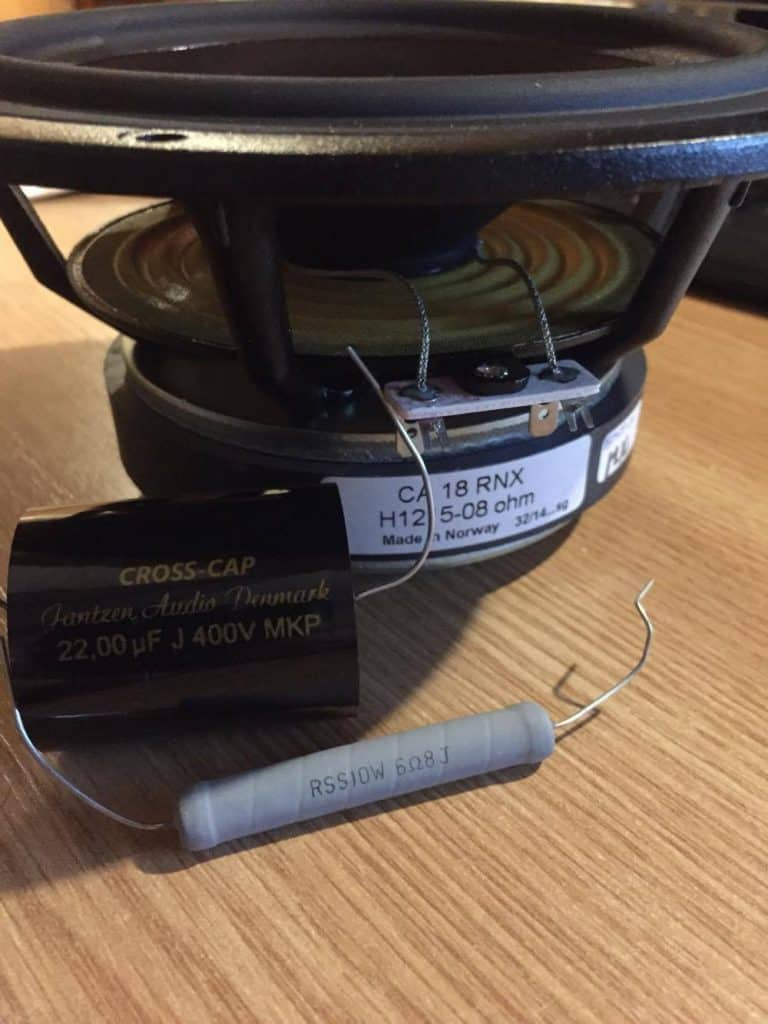After setting up the impedance equalization circuit, I did another impedance sweep, to see what the Zobel network has done to the impedance curve.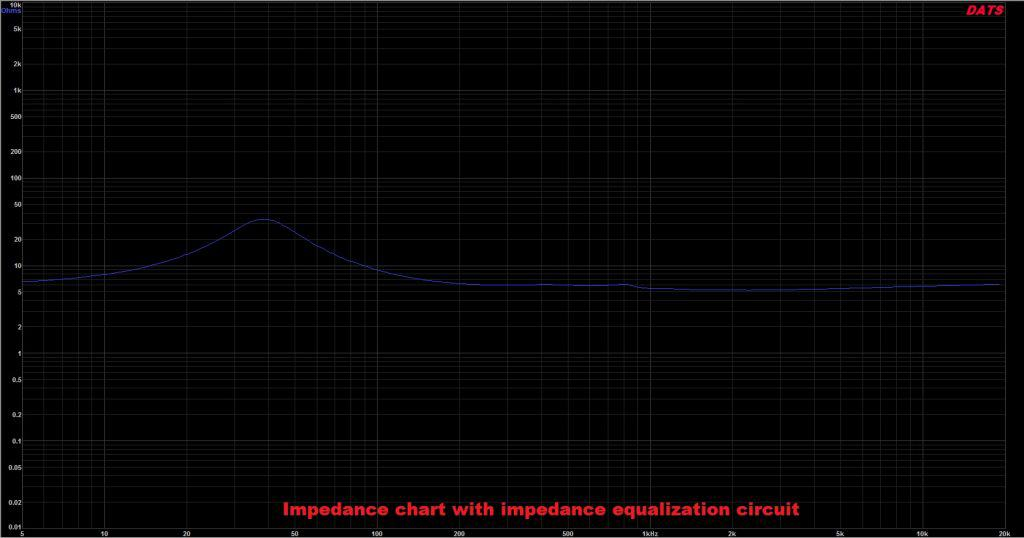Finally, the impedance has flattened, and it’s nice and smooth. You are probably wondering: What about the hump at resonance? There is no concern regarding the impedance spike at resonance. This is because we are making a low pass filter for a mid-bass driver. In conclusion, we are filtering the high frequencies, so the hump at 40 Hz doesn’t affect the crossover network.  However, if we are talking about a tweeter, that’s a different story. When making a high pass filter for a tweeter, the spike at resonance is something to worry about. Anyway, we will talk about how to flatten tweeter’s impedance on a different occasion.

### Conclusion

The Zobel network / circuit is a good choice when it comes to designing passive crossover networks. Some people disregard it, as it can require a rather large capacitor, which are quite expensive. Nevertheless, it’s very easy to design and implement. The hard part is when you start to tinker with the actual crossover network.

#### References

1. Loudspeaker Design Cookbook 7th Edition by Vance Dickason (Audio Amateur Pubns, 2005).
2. Electrical Networks by A. Henderson (Arnold, 2014).